# Methods and formulas for the random effect predictions for Stability Study for random batches

Select the method or formula of your choice.

## Best linear unbiased predictors (BLUP)

The BLUP are the predicted values of the random terms in the model. Recall the general form of the mixed model:
The vector that produces the BLUP estimates is:

where

For further details on the notation, go to the Methods section.

## Standard deviation of BLUP

The estimated standard deviations are the square roots of the diagonals of this matrix:

where

### Notation

TermDescription
Zithe n x mi matrix of known codings for the ith random effect in the model
Z'the transpose of Z
ythe vector of the response values
Xthe design matrix
bthe estimated coefficients for the fixed effects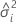the variance component of the ith random factor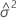the variance component for error
Imthe identity matrix with m rows and columns

## Degrees of freedom for BLUP

The degrees of freedom for the test of theBLUP component are:

where

### Notation

TermDescription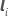a vector with value 1 at therow and 0 elsewhere with dimension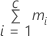Wthe asymptotic variance-covariance matrix of the variance component estimates

For further details on the notation, go to the Methods section.

## p-value for BLUP

The two-sided p-value for the null hypothesis that a Best Linear Unbiased Predictor (BLUP) equals 0 is:

### Notation

TermDescription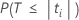The probability that under the null hypothesis T is less than the absolute value of the calculated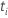. Here, T follows a t-distribution with df degrees of freedom.The t value for theBLUP.
By using this site you agree to the use of cookies for analytics and personalized content.  Read our policy